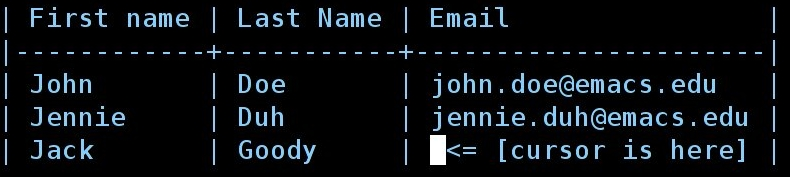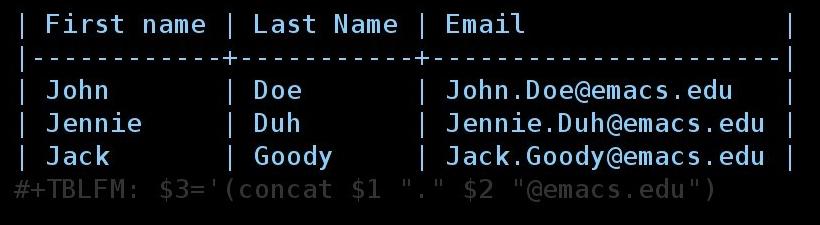Support via Liberapay, GitHub or PayPal

# Org as a spreadsheet system: using Emacs lisp as formulas

## Introduction

This tutorial explains how to use Emacs Lisp as formulas in Org tables. If you want a general tutorial on how to use Org as a spreadsheet system, read this tutorial. You can also check the complete Org documentation on this topic1.

## Example 1: manipulate cells

Here is a simple table:

First name Last Name Email
John Doe john.doe@emacs.edu
Jennie Duh jennie.duh@emacs.edu

You easily notice the pattern `[firstname].[lastname]@emacs.edu` for the third column. Given a first name and a last name, it should be easy to compute the result for the "Email" column.

Okay, let's do it then. First put your cursor in the third column:Now type `C-c }` to display the table's coordinates.

For each row, you want to concatenate the content of the first column (accessed with `\$1`) to a string with a dot (".") to the cell of the second column (accessed with `\$2`) and to the string "@emacs.edu". In Emacs Lisp, you would write this:

```'(concat \$1 "." \$2 "@emacs.edu")
```

Now copy this formula, type `C-c =` in the bottom right field to insert a column formula2 and copy the formula here. Hitting `RET` will immediately insert the result in this field (`Jack.Goody@emacs.edu`) and add the `#+TBLFM` line at the bottom of the table.

Caveat: beware of the initial quote: the formula is the expression itself, not its value. This expression will only have a meaning when the `\$1` and `\$2` references will be replaced by the right strings, an the expression will then by applied as a formula by pressing `C-c C-c` on `#+TBLFM`.

So you now have this table:At the time the formula is evaluated, the references `\$1` and `\$2` are interpreted and replaced by the values of these cells as strings: you don't need to enclose `\$1` with quotes ("\$1").

If you want to force the references (`\$1` and `\$2`) to be interpreted as numbers, add the flag `;N` at the end of the Emacs lisp expression. See for example this table

First name Last Name Maths French Mean
John Doe 12 16 14
Jennie Duh 15 9 12

where we used this formula for the fifth column:

```#+TBLFM: \$5='(/ (+ \$3 \$4) 2);N
```

As an exercise, try to find Emacs lisp formula for the fifth column in this table:

First name Last Name Maths French Mean
John Doe 12 16 John: 14
Jennie Duh 15 9 Jennie: 12

All you have is the values of the first four columns. (Hint: check the Emacs lisp functions `string-to-number` and `number-to-string`.)

Solution: we cannot use the `;N` flag because it will force interpretation of the cells as numbers, and if we do so, we will not be able to access the value of cells in the first row. So one idea is to use `string-to-number` and `number-to-string` this way:

```#+TBLFM: \$5='(concat \$1 ": " (number-to-string (/ (+ (string-to-number \$3) (string-to-number \$4)) 2)))
```

Another idea is to use the `;L` flag: instead of using interpreting cells as strings or numbers, it inserts their content literally in the Emacs lisp expression. So the formula above can safely be replaced by this more condensed one:

```#+TBLFM: \$5='(concat "\$1" ": " (number-to-string (/ (+ \$3 \$4) 2)));L
```

Note the double-quotes around "\$1": that's because inserting a first name literally would mean "it is an Emacs lisp symbol". So, when using the `;L` flag, adding double-quotes makes sure the reference is interpreted as a string.

We're done!

## Example 2: manipulating ranges

Suppose now we have this table

Col1 Col2 Col3 Col4 Col5
? ? in Col1 and Col2 (no duplicates) only in Col1 only in Col2
? ?
? ?

`Col1` and `Col2` contain strings.

We want the first cell of the third column to contain a string with all the strings that are in both `Col1` and `Col2`, with no duplicates. `Col4` contains strings that are only in `Col1` (and not in `Col2`) whereas `Col5` contains strings that are only in `Col2` (and not in `Col1`).

How to automate this with an Emacs lisp formula?

Let's first figure out the result we want for a simple table:

Col1 Col2 Col3 Col4 Col5
a a a b c d c d
a b
b a
c d

Now let's get the values of the first column starting from the second row.

The "a" on the top left cell can be accessed through the reference `@2\$1`. The "c" on the bottom left cell can be accessed through the reference `@5\$1`. The range of cells can then be accessed with `@2\$1..@5\$1`.

Let's add this range in the first cell of `Col3`:

Col1 Col2 Col3 Col4 Col5
a a a a b c c d
a b
b a
c d

Here is the formula:

```#+TBLFM: @2\$3='(mapconcat 'identity (list @2\$1..@5\$1) " ")
```

When interpreted, the range `@2\$1..@5\$1` is replaced by the values of the cells, separated with a space. So `(list @2\$1..@5\$1)` becomes ```(list "a" "a" "b" "c")``` and the whole formula becomes

```'(mapconcat 'identity (list "a" "a" "b" "c") " ")
```

which basically means "Concatenate elements of ("a" "a" "b" "c") and add a single space between each of them".

Let's generalize and say that we don't know how many rows our table contains. The range `@2\$1..@5\$1` becomes `@2\$1..@>\$1` where `@>` means "the last row" and `@>\$1` "the last row in the first column".

Remember: we want the third column to contain a string with all the strings that are in both `Col1` and `Col2`, with no duplicates. Let's first list all values from `Col1` and `Col2` with `(list @2\$1..@>\$1 @2\$2..@>\$2)`, then delete duplicates like this `(delete-dups (list @2\$1..@>\$1 @2\$2..@>\$2))` then put this expression in the one we already have above.

```#+TBLFM: @2\$3='(mapconcat 'identity (delete-dups (list @2\$1..@>\$1 @2\$2..@>\$2)) " ")
```
Col1 Col2 Col3 Col4 Col5
a a a b c d ? ?
a b
b a
c d

Okay. Now that you know how to manipulate ranges, you can replace the "?" with the right formulas… remember: `Col4` contains strings that are only in `Col1` and not in `Col2`, whereas `Col5` contains strings that are only in `Col2` and not in `Col1`. (Hint: you can write your own functions and use them in an Emacs lisp formula.)

Don't forget that you can edit a table's formulas with by hitting C-c ' anywhere on the table: it will open the formulas editor, which highlights references that the cursor is on (both in the formulas editor and in the table). The formulas editor is really handy when you need to check that your references are correct. Also, hitting `TAB` on a formulas in this editor will pretty-print the formula, which helps editing a lot !

## Conclusion

Please check the Org manual for (condensed but accurate and up to date) information on using Lisp as formulas: see the manual online or as an Info page.

## Footnotes:

1

If you are reading this tutorial from within Emacs, go to the spreadsheet section of the manual by clicking on this link: the spreadsheet.

2

Column formulas apply to the whole column, whereas Field formulas only apply to the current field. Field formulas can be inserted by pressing `C-u C-c =` in a field.

Documentation from the orgmode.org/worg/ website (either in its HTML format or in its Org format) is licensed under the GNU Free Documentation License version 1.3 or later. The code examples and css stylesheets are licensed under the GNU General Public License v3 or later.